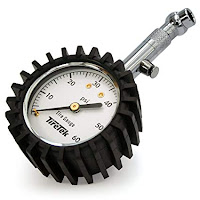## Thursday, November 7, 2019

### Why Tire Pressure Drops in the Fall.

The other day I saw something click-baity on Facebook (I think) that said "The science behind why tire pressure drops in the fall." And I said "That's easy: PV=nRT."

If you're saying "huh?" you never took freshman chemistry.

The equation PV=nRT is the "ideal gas law." An ideal gas is one that follow this formula exactly. A lot of gases come close and for those who don't, there's fudge factor called "fugacity." But we'll sick with the ideal gas law because air operates pretty closely to it (its fugacity almost equals 1).

But what does PV=nRT mean?

P = pressure. This is measured in psi (pounds per square inch) for tires but pascals in freshman chemistry class. Those are the "SI" units of pressure

V = volume, measured in liters in freshman chemistry class/SI units.

n = the amount of gas measure in moles. What's a mole? A mole is  6.0221409e+23 atoms or molecules. Technically, it should be written like this:

but that's difficult in a blog post.  6.0221409e+23 is the computer way to write scientific notation.

(That's 60221409 followed by 15 zeros or approximately 60 sextillion atoms. See below.) One mole of an element will weigh it's "atomic mass" in grams. If you look on a periodic table, Hydrogen's atomic mass is very close to 1. So one mole of hydrogen equals about one gram of hydrogen.

R= is Boyle's Constant and is equal to 8.314 joules/mole. Don't worry about that.

And T = temperature.

So, again, for our purposes, V is a constant (the volume inside the tire stays the same). "n" is a constant (you're not adding or taking out any air). And R is always a constant. So a little algebra and you get P=Tk (where "k" is a constant).

So if T (temperature) goes down, what happens to P (pressure)? The pressure has to go down proportional to k.

And what happens in the Fall? Ambient temperatures drop.

But wait! The math says that the pressure drops when the temperature drops. But why does that happen? The math is a model of the real world, not the real world. (See below for a math joke.)

What is temperature? It is a measurement of the vibration of atoms and molecules. The higher the temperature, the more they vibrate. At lower temperatures, they vibrate less. At absolute zero, all vibration stops (this is a concept, the energy to reach absolute zero is thought to be infinite).

As we all know (I hope), molecules are atoms that are connected together. Most of your air is a molecule with two nitrogen atoms.

So think of a balloon (tires are sort of like balloons). You blow air into it and it expands. Why? Because the atoms and (more likely) molecules of air are banging against the inside wall of the balloon more than the atoms and molecules are banging against the outside of the balloon. This is because of pressure, not temperature. You blew air into it, increasing the pressure of the air inside the balloon.

Now, if the balloon gets hotter, the molecules and atoms vibrate faster and hit the rubber of the balloon harder, causing it to expand. If the balloon cools off, the molecules and atoms hit it less hard and the balloon shrinks.Same thing happens in your tires, except the volume stays pretty much the same (unless the pressure really drops) so when the temperature drops, the pressure drops. In the spring, the opposite happens. It warms up, and the tire pressure goes up.

This (and the fact tires tend to lose air over time, especially Goodyear tires) is why I strongly recommend checking tire pressure at least once per month. Buy a good tire pressure gauge and check that pressure. If it's too low, you'll need to add air. Your tire pressure gauge doesn't need to be fancy, but I'd stay away from the stick kind.

So there you have it, the science behind why tire pressure drops in the fall.

(That number of atoms in a mole is 60,221,409,000,000,000,000,000.)

Math Joke
Physicists think the real world approximates the math
Engineers think the math approximates the real world.
Mathematicians never make the connection.